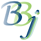# SQL — Time and Date Functions

The following time and date functions specified in the ODBC 3.51 and JDBC 2.0 specifications are supported by the BASIS DBMS.

Arguments denoted as date_exp can be the name of a column (of SQL_DATE, Julian, or OEM date type) or a date literal (constant).

Function

Description

CURDATE()

Returns the current date as a standard SQL DATE type value. BBj language-specific note: When returning SQL DATE values to BBj through SQLFETCH, the string template used to define the record contains a type I(4) field containing the date value as a Julian number.

CURTIME()

Returns the current time as a standard SQL TIME type value. BBj language-specific note: When returning SQL TIME values to BBj through SQLFETCH, the string template used to define the record contains a type C(23) field containing the time value as a string in the format hh:mm:ss.

DAYNAME(date_exp)

Returns a character string containing the name of the day of the week (e.g., Sunday) for the day portion of date_exp.

DAYOFMONTH(date_exp)

Returns the day of the month in date_exp as an integer value in the range of 1-31.

DAYOFWEEK(date_exp)

Returns the day of the week in date_exp as an integer value in the range of 1-7, where 1 represents Sunday.

DAYOFYEAR(date_exp)

Returns the day of the year in date_exp as an integer value in the range of 1-366.

DAYSINMONTH(date_exp)

Returns the number of days in the month specified. Function takes a standard date or date string, but it also accepts a modified date string that includes only year and month in either YYYYMM or YYYY-MM format.

DAYSINYEAR(date_exp)

Returns the number of days in the year specified. Function takes a standard date or date string, but it also accepts a full four digit year as a numeric or string value.  Function automatically takes into account leap years.

HOUR(time_exp)

Returns the hour based on the hour field in time_exp as an integer value in the range of 0-23.

ISDATE(char_exp)

Returns 1 if the parameter value can be parsed as a date value in the standard SQL DATE format of yyyy-mm-dd. Returns 0 if it is not in this format. This function will also return 1 for values in the standard TIMESTAMP format yyyy-mm-dd hh:MM:ss. If the parameter value is a DATE type expression, it will always return 1.

ISNUMERIC(char_exp)

Returns 1 if the parameter value can be parsed as a number or 0 if not. This accepts digits, decimal point, and sign characters. If the parameter is a numeric type expression (INTEGER, FLOAT, DECIMAL, etc.) it will always return 1.

MINUTE(time_exp)

Returns the minute based on the minute field in time_exp as an integer value in the range of 0-59.

MONTH(date_exp)

Returns the month in date_exp as an integer value in the range of 1-12.

MONTHNAME(date_exp)

Returns a character string containing the name of the month (e.g., January through December) for the month portion of date_exp.

NOW()

Returns the current date and time as a timestamp value.

QUARTER(date_exp)

Returns the quarter in date_exp as an integer value in range of 1-4, where 1 represents January 1 through March 31.

SECOND(time_exp)

Returns the second based on the second field in time_exp as an integer value in the range of 0-59.

Returns the timestamp calculated by adding integer_exp intervals of type interval to timestamp_exp. Possible values for the interval are: SQL_TSI_FRAC_SECOND, SQL_TSI_SECOND, SQL_TSI_MINUTE, SQL_TSI_HOUR, SQL_TSI_DAY, SQL_TSI_WEEK, SQL_TSI_MONTH, SQL_TSI_QUARTER or SQL_TSI_YEAR.

USE_DATE_FORMAT(raw_data, bbj_date_format)

In BBj 11.0 and higher, this function uses the specified bbj_date_format to process the data in raw_data, returning a valid SQL DATE or TIMESTAMP value depending on the type of date format. Valid values (case sensitive) for bbj_date_format include: ADP, AON, AON3_CYYMMDD, CITI, CYYMMDD, DayMonthYear,  DISC, Julian, Julian_Time, MAI, Millisecond, MonthDayYear, SOA, SQLDate, SSI, SSIJ and YMD8.

WEEK(date_exp)

Returns the week as an integer value in range 1-52, where '1998-01-16' would return 3.

YEAR(date_exp)

Returns the year in date_exp as an integer value.

## Example

Select ship_date, Dayname(ship_date), Monthname(ship_date) from  order_header

The following three types of arguments are allowed in the Time/Date functions:

CURTIME() or
Time Constant

OEM/Julian Date or
Date Constant or
CURDATE()

Timestamp field or
NOW()

HOUR

Yes

Yes

MINUTE

Yes

Yes

SECOND

Yes

Yes

YEAR

Yes

Yes

MONTH

Yes

Yes

DAYOFWEEK

Yes

Yes

QUARTER

Yes

Yes

DAYNAME

Yes

Yes

DAYOFMONTH

Yes

Yes

DAYOFYEAR

Yes

Yes

MONTHNAME

Yes

Yes